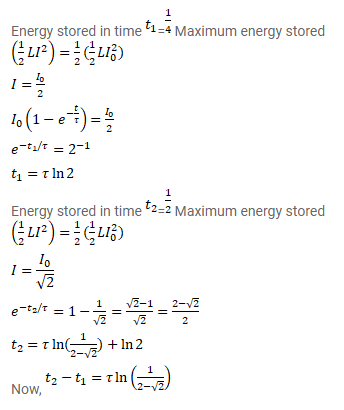# A coil having an inductance L and a resistance R is

Question:

A coil having an inductance $L$ and a resistance $R$ is connected to a battery of emf $\varepsilon$. Find the time taken for the magnetic energy stored in the circuit to change from one fourth of the steady-state value to half of the steady-state value.

Solution: